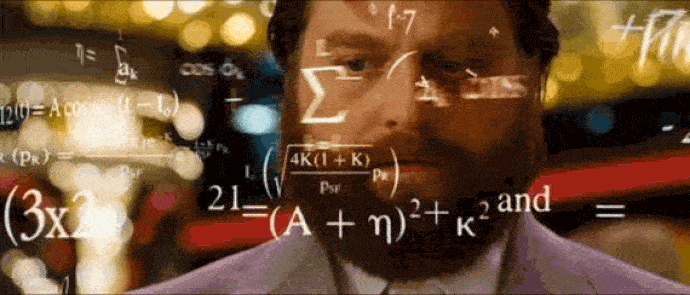## DEV Community is a community of 846,223 amazing developers

We're a place where coders share, stay up-to-date and grow their careers.# Sorting algorithms: JavaScript - Bubble Sort🚀

* 🤓INTRODUCTION
* 🥚WHY SORTING ALGORITHM
* 🎈WHAT IS BUBBLE SORT
* 🛠IMPLEMENTATION
* 👩🏻‍💻CODE
* 🙏THANK YOU

## 🤓 INTRODUCTION

Hello, my dear coders! I hope you are all having a wonderful time coding, enjoying your life. In this blog series, we will discuss sorting algorithms and we will implement those algorithms using javascript. Connect with me via Twitter or LinkedIn

Algorithms are a very important part of programming and are a part of job interviews.

Let's dive in, and prepare you for the next interview! 🚀## 🥚 WHY SORTING ALGORITHM

There are several algorithms that solve the following sorting problem.

Input: A sequence of n numbers (a1,a2,...,an)
Output: A permutation (reordering) of the input sequence

The input sequence is usually an n-element array, although it may be represented in some other fashion, such as a linked list.

### THE STRUCTURE OF THE DATA

In practice, the numbers to be sorted are rarely isolated values. Each is usually part of a collection of data called a record. Each record contains a key, which is the value to be sorted. The remainder of the record consists of satellite data, which are usually carried around with the key.

When the sorting algorithm permutes the keys, it must permute the satellite data as well. If each record includes a large amount of satellite data, we often permute an array of pointers to the records rather than the records themselves.

### WHY SORTING?

Many computer scientists consider sorting to be the most fundamental problem in the study of algorithms.

There are several reasons:

• Sometimes an application inherently needs to sort information. For example, in order to prepare customer statements, banks need to sort checks by check number.
• Algorithms often use sorting as a key subroutine. For example, a program that renders graphical objects which are layered on top of each other might have to sort the objects according to an “above” relation so that it can draw these objects from bottom to top. We shall see numerous algorithms in this text that use sorting as a subroutine.
• We can draw from among a wide variety of sorting algorithms, and they employ a rich set of techniques. In fact, many important techniques used throughout algorithm design appear in the body of sorting algorithms that have been developed over the years. In this way, sorting is also a problem of historical interest.
• Many engineering issues come to the fore when implementing sorting algorithms. The fastest sorting program for a particular situation may depend on many factors, such as prior knowledge about the keys and satellite data, the memory hierarchy (caches and virtual memory) of the host computer, and the software environment.

## 🎈 BUBBLE SORT ALGORITHM**The Bubble Sort algorithm compares elements two by two, and an element with a greater value moves on, and just like that in the first iteration an element with the smallest value "emerges" in the first position.

## 🛠 IMPLEMENTATIONSo if we start with an array [11, 10, 2, 5, 7], after applying the bubble sort algorithm we will get an array [2, 5, 7, 10, 11].

## 👩🏻‍💻 CODE

``````function bubble_sort_algorithm(array) {
const t0 = performance.now(); //this is just for calculating time, ignore it
const length = array.length; //get the length of an array
for (let i = 0; i < length; i++) {
//Loop 1: go from 0 to the length - 1
for (let j = length - 1; j > i; j--) {
//Loop 2: go from length - 1, while larger than i, and decrement j
if (array[j] < array[j - 1]) {
//check for an element value if current element smaller than the previous element
let temporary = array[j]; //do the swap
array[j] = array[j - 1];
array[j - 1] = temporary;
}
}
}
const t1 = performance.now();
console.log(`Time spent executing the function - \${t1 - t0} miliseconds`);
return array;
}

let array = [11, 10, 2, 5, 7];
console.log(bubble_sort_algorithm(array));
``````

## 👨🏻‍🔬 COMPLEXITY OF THE BUBBLE SORT ALGORITHM

The complexity of the bubble sort algorithm, in both worst and best case, is always Big O of n2

References:
School notes...
School books...